Dice
 What are the Dice?  There are 30 Dice Appearance of  the Standard Die Four Puzzles:  ...Guessing the Sum ...Cube Puzzle ...Rubik's Dice ...Sum Problem More Dice Shapes Marked Dice The Computer Plays the Dice Dice on the Internet References . ..
 To the Main Page   "Mathematische Basteleien"
What are the Dice?
 ...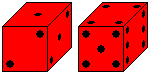... The dice have 21 points. The numbers 1 to 6 appear on the six sides of a cube. - You throw the dice by hand or by a leather dice cup. You turn the cup upside down and you let the dice roll to a standstill.
It is by chance which number appears on top. This is the attraction of throwing the dice.
The dice are used in many board games. They often determine the playing. They give the game unexpected turns and add excitement to the games.

There are 30 Dice top
 ...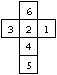... If you give the numbers 1, 2, 3, 4, 5, and 6 and form all permutations of the six numbers, you get 1*2*3*4*5*6=6!=720 cubes. Now there are also those dice among them, which are the same, because you can get them by turning around 13 axes. There are 24 turnings.  Hence you only have 720:24 =30 different cubes.
The following drawing illustrates this. (The numbers under the cubes give you the number of turnings.)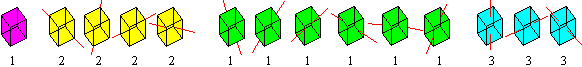Appearance of  the Standard Die    top
The die has six sides for six numbers. If you try all possible ways to spread them out, you get 30 dice. - Every die has its reflection. You can find the possibilities if you reverse the numbers beside the 1.
 ...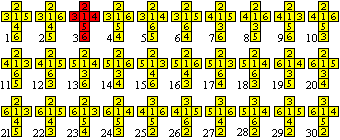... You can mark every die by a number with six digits: Start with 1, go upwards, go around 1 counter clockwise and add the number that appears opposite the number 1 on the die. - The red die has 123546 for instance. The dice on the left are ordered by these numbers.
You find more about the number 30 at my web page Macmahons Coloured Cubes.

There is only the red die in the Western World.
 ...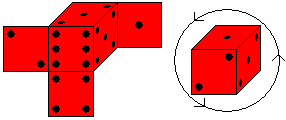... This die has two characteristics:  >The sides opposite each other always add to 7.  >If you look at the corner with the numbers 1, 2, and 3 they are arranged anticounter clockwise.
Most of the dice makers follow these rules.

 ...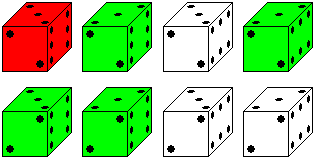...... There is a way the dice can be different. There are two ways of placing  the points of number 2, 3, or 6, which change into themselves by turning through 180°. This leads to eight pictures.  The red die is the most frequent. But I also found the green ones.

Four puzzles with dice will follow now.

Guessing the Sumtop
 ...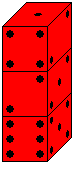... Build a tower of several dice.  Is it possible to find the sum of the points of the hidden horizontal cube sides? In this case the tower is formed by three dice. Five surfaces would be counted: The under side of the top die, and the top and bottom sides of the two lower dice.  Solution: You take 1 at the top and count the number of the lower cubes. Number 6 is opposite the 1 side. You add 7 for each die. The sum is 6+2x7=20.

Cube Puzzle    top
 ...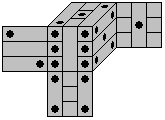... You can make a nice cube puzzle, if you cut the die in nine bars.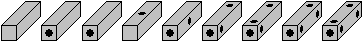The vertical bars are in the middle layer, the horizontal ones on the outside. Then the puzzle is more interesting.
 ...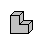... You can also form a 3x3x3-cube with 9 V-Triominos (drawing) or with Soma cubes. Draw points on them.

Rubik's Dice  top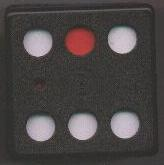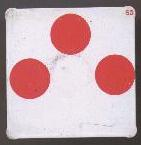Rubik's dice is a black plastic dice 7cm x 7cm x 7cm with circular holes instead of points. There are seven white squared tiles with red circles, which you can lay inside the cube. The tiles directly lying at the holes stick to walls inside. You can remove them with two connected sticks. The tiles close the holes in red or in white. You have found a solution, when all points have become white.

You will soon notice, that you can`t solve the puzzle by trying. There are too many possibilities with seven tiles for closing the holes. Moving the tiles is difficult, too.

Act like this: You can look inside the cube and can recognize the patterns of both sides of the tiles. Draw them. The tiles show a number with two digits on the top right (meaning?), which can be used as names. There is the following image: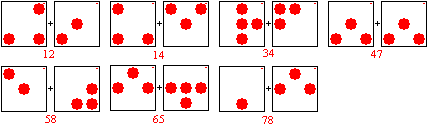Now the solution is simple. If you like, don't read further and solve the puzzle yourself...

Solution:
Tile 78 (left side) only fits on the six, then tile 65 (left side) only fits on the five, then tile 34 (left side) only fits on the four, then tile 58 (right side) only fits on the three, then tile 12 (right side) only fits on the two, and then tile 14 (left side) only fits on the one. Tile 47 is useless.
There is only one solution.

In the meantime I opened the cube with a saw :-( to scan one side of a tile. Besides I was curious to see how the tiles stick inside. The tiles are made of iron. They are held by six ring magnets. They are inside the cube in the centres. You can see their contours from outside.

Sum Problem    top
 ...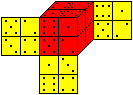... Give eight dice. Build a 2x2x2 cube, so that the sum of the points on each side is the same. Here is one of  20 736 solutions with the sum 14.
You find more at the German magazine <bild der Wissenschaft> 3-1980.

More Dice Shapes    top
The cube belongs to the five Platonic solids. These are solids, which are only formed by regular polygons. They are called  tetrahedron, hexahedron (cube), octahedron, dodecahedron, and icosahedron.

You can build dice after their model.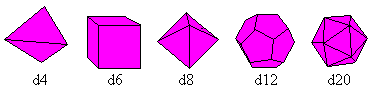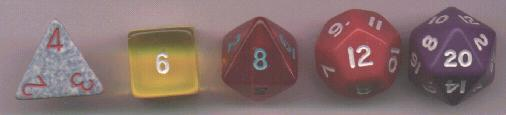You can find 4,6, 8,12 or 20 numbers by throwing the dice. The number at the top counts. The tetrahedron is an exception. The hidden number counts or the one, that is on top as seen in the scan.

I found more interesting solids, which are possible to play the dice from the mathematical view.  They are convex, are formed by congruent polygons and have the same position related to the centre of gravity.  ("Properties of Dice", URL below).

There are more dice forms. I chose two of them.
 ...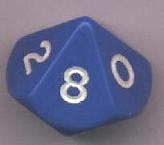... The die d10 is interesting. It has the shape of a double pyramid with a pentagon as the mutual base. It shows the numbers 0 to 9, the digits of the decimal system. So you can find random numbers with several digits by several dice of this kind.

 ...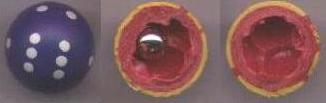.... The die in ball form is funny:  There is an iron ball inside the die, which only rest in one of the six hollows. So the points lie at the top.

 ...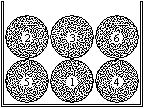... There are many possibilities to find random numbers. You can lay six balls marked with number 1 to 6 in a box similar to the national lottery. You can mix them and then take one without looking at. The chosen number is the random number. If you play the "dice" a second time, you must put back the ball in the box first.  This is the normal model for a die in mathematics to classify the die in a theory.

In spite of the inflation of dice forms:  The normal d6 is and will stay the standard die.
>The number 6 is not too small and not too large. You can play well with six random numbers.
>The normal die rolls best, especially if the corners are rounded. It is common.
>You can make them easily, because the cube sides are perpendicular to each other.

Marked Dice    top

You call dice, which show some numbers more frequently than others, marked dice.

You can manipulate the normal die in different ways.

> The dice don`t show all six different numbers, some are missing. You always see three sides of the dice from one direction. Therefore three numbers can be missing.
> You can slightly change the shape of the dice. You can irregularly round off the corners, the areas of a side can be different.
> You can manipulate the dice inside. If there is a metal piece inside the dice, but not symmetrically, the dice will stop rolling, so the heavy piece will lay at the bottom. - A hollow space will be at the top.
> You can prepare the surface of a cube side, so it will stop on this side.
> You can use magnetic power, even switched on by radio control.

The Computer Plays the Dice    top
You can simulate the dice with the computer. There is a random generator in all programming languages (i.e. Visual Basic), which produce random numbers between 0 and 1 (A=RND).
 ...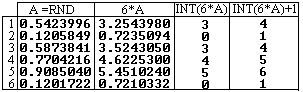... You multiply these numbers by 6, cut off the decimals with the function INT and add 1. This is shown with six examples on the left.  You get the random numbers 4, 1, 4, 5 ,6, and 1.

It is easy to throw the dice 6000x this way.
 ...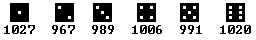... The frequency table shows that the numbers from 1 to 6 appear nearly equally, about 1000x.
This also predicts the probability theory. The probability finding a number (i.e. three) is 1/6 (=1000/6000). This result is better and better, if  the number of throwing the dice is bigger. On the other hand a good random generator must not distribute the numbers too regularly. This is the difficulty with programming a good random generator.

A probability statement like 1/6 is of no use for a single throwing. You don`t know which number will appear next. Each throwing is independent from the one before.

Dice on the Internet top

English

Kevin Cook
Collection of Dice

Eric W. Weisstein (MathWorld)
Dice

Klaus Æ. Mogensen
Properties of Dice

Leo van der Heijdt
Welcome to the Homepage of  DICE  Collector (Dutch / English)

Wikipedia
Dice

German

Faroul
Würfel

Wikipedia
Spielwürfel

References (German)  top
(1) Robert E.Lembke, Michael Schiff: Das große Haus- und Familienbuch der Spiele, Frankfurt a. M. 1970
(2) Martin Gardner: Mathematische Hexereien, Berlin 1988
(3) Rüdiger Thiele, Konrad Haase: Der verzauberte Raum, Leipzig 1991
(4) Reiner Knizia: Das große Buch der Würfelspiele (124 Spiele), München 2000

Gail from Oregon Coast, thank you for supporting me in my translation.

Feedback: Email address on my main page